Test: Combinational Logic Circuits - 1

# Test: Combinational Logic Circuits - 1

Test Description

## 20 Questions MCQ Test GATE ECE (Electronics) 2023 Mock Test Series | Test: Combinational Logic Circuits - 1

Test: Combinational Logic Circuits - 1 for Electronics and Communication Engineering (ECE) 2023 is part of GATE ECE (Electronics) 2023 Mock Test Series preparation. The Test: Combinational Logic Circuits - 1 questions and answers have been prepared according to the Electronics and Communication Engineering (ECE) exam syllabus.The Test: Combinational Logic Circuits - 1 MCQs are made for Electronics and Communication Engineering (ECE) 2023 Exam. Find important definitions, questions, notes, meanings, examples, exercises, MCQs and online tests for Test: Combinational Logic Circuits - 1 below.
Solutions of Test: Combinational Logic Circuits - 1 questions in English are available as part of our GATE ECE (Electronics) 2023 Mock Test Series for Electronics and Communication Engineering (ECE) & Test: Combinational Logic Circuits - 1 solutions in Hindi for GATE ECE (Electronics) 2023 Mock Test Series course. Download more important topics, notes, lectures and mock test series for Electronics and Communication Engineering (ECE) Exam by signing up for free. Attempt Test: Combinational Logic Circuits - 1 | 20 questions in 60 minutes | Mock test for Electronics and Communication Engineering (ECE) preparation | Free important questions MCQ to study GATE ECE (Electronics) 2023 Mock Test Series for Electronics and Communication Engineering (ECE) Exam | Download free PDF with solutions
 1 Crore+ students have signed up on EduRev. Have you?
Test: Combinational Logic Circuits - 1 - Question 1

### A switching function of four variable, f (w, x y, z) is to equal the product of two other function f1 and f2, of the same variable f = f1f2 . The function f and f1 are as follows : f = ∑m(4,7,15) f = ∑m(0,1,2, 3, 4,7, 8,9,10,11,15) Que: The number of full specified function, that will satisfy the given condition, is

Detailed Solution for Test: Combinational Logic Circuits - 1 - Question 1

f = ∑m(4,7,15)

f1 = ∑m(0,1,2, 3, 4,7, 8,9,10,11,15)

f2 = ∑m(4,7,15) + ∑dc(5, 6, 12, 13, 14)

There are 5 don't care condition. So 25 = 32 different functions f2

Test: Combinational Logic Circuits - 1 - Question 2

### A switching function of four variable, f (w, x y, z) is to equal the product of two other function f1 and f2, of the same variable f = f1f2 . The function f and f1 are as follows : f = ∑m(4,7,15) f1 = ∑m(0,1,2, 3, 4,7, 8,9,10,11,15) Que: The simplest function for f2 is

Detailed Solution for Test: Combinational Logic Circuits - 1 - Question 2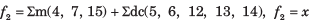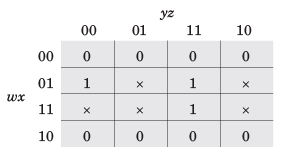Test: Combinational Logic Circuits - 1 - Question 3

### A four-variable switching function has minterms m6 and m9. If the literals in these minterms are complemented, the corresponding minterm numbers are

Detailed Solution for Test: Combinational Logic Circuits - 1 - Question 3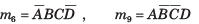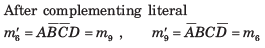Test: Combinational Logic Circuits - 1 - Question 4

The minimum function that can detect a “divisible by 3’’ 8421 BCD code digit (representation D8 D4 D2 D1 ) is given by

Detailed Solution for Test: Combinational Logic Circuits - 1 - Question 4

0, 3, 6 and 9 are divisible by 3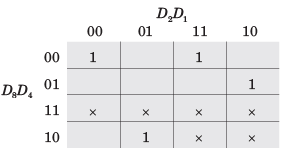f =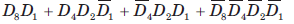Test: Combinational Logic Circuits - 1 - Question 5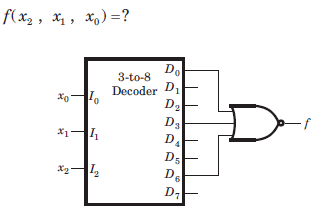Detailed Solution for Test: Combinational Logic Circuits - 1 - Question 5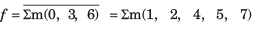Test: Combinational Logic Circuits - 1 - Question 6

For a binary half subtractor having two input A and B, the correct set of logical expressions for the outputs D = (A - B) and X (borrow) are

Detailed Solution for Test: Combinational Logic Circuits - 1 - Question 6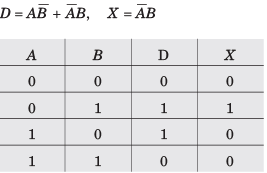Test: Combinational Logic Circuits - 1 - Question 7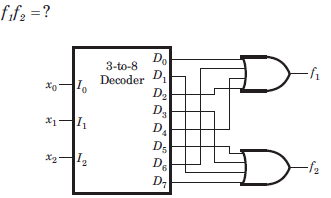Detailed Solution for Test: Combinational Logic Circuits - 1 - Question 7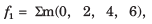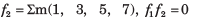Test: Combinational Logic Circuits - 1 - Question 8

What type of logic circuit is represented by the figure shown below?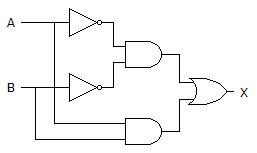Detailed Solution for Test: Combinational Logic Circuits - 1 - Question 8

After solving the circuit we get (A’B’)+AB as output, which is XNOR operation. Thus, it will produce 1 when inputs are even number of 1s or all 0s, and produce 0 when input is odd number of 1s.

Test: Combinational Logic Circuits - 1 - Question 9

The building block shown in fig. is a active high output decoder.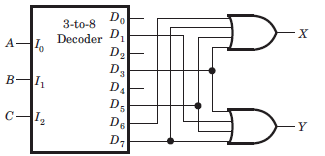Que: The output X is

Detailed Solution for Test: Combinational Logic Circuits - 1 - Question 9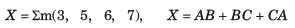Test: Combinational Logic Circuits - 1 - Question 10

The building block shown in fig. is a active high output decoder.Que: The output Y is

Detailed Solution for Test: Combinational Logic Circuits - 1 - Question 10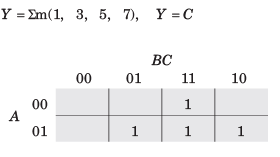Test: Combinational Logic Circuits - 1 - Question 11

A logic circuit consist of two 2 x 4 decoder as shown in fig.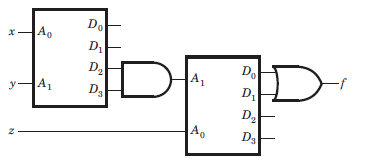The output of decoder are as follow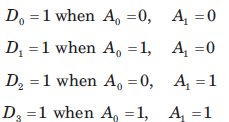The value of f ( x, y, z) is

Detailed Solution for Test: Combinational Logic Circuits - 1 - Question 11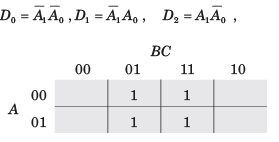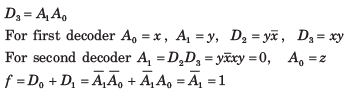Test: Combinational Logic Circuits - 1 - Question 12

Number of 2 × 1 Multiplexers are required to implement 64 × 1 Multiplexers

Detailed Solution for Test: Combinational Logic Circuits - 1 - Question 12

A 64 × 1 multiplexer has 64 inputs so if we use 2 × 1 multiplexers 32 are needed in the first stage for 64 inputs, the output of these 32 multiplexers are connected to inputs of 16 multiplexers in the second stage.

Similarly, in third stage, 8 (2 × 1) multiplexers are used, in fourth stage 4 are used and finally 2 (2 × 1) multiplexers in the fifth stage, 1 in the sixth stage.

Total 2 × 1 multiplexers needed are 32 + 16 + 8 + 4 + 2 + 1 = 63

Test: Combinational Logic Circuits - 1 - Question 13

How many 3-line-to-8-line decoders are required for a 1-of-32 decoder?

Test: Combinational Logic Circuits - 1 - Question 14

Number of 2 × 1 Multiplexers are required to implement 64 × 1 Multiplexers

Detailed Solution for Test: Combinational Logic Circuits - 1 - Question 14

A 64 × 1 multiplexer has 64 inputs so if we use 2 × 1 multiplexers 32 are needed in the first stage for 64 inputs, the output of these 32 multiplexers are connected to inputs of 16 multiplexers in the second stage.

Similarly, in third stage, 8 (2 × 1) multiplexers are used, in fourth stage 4 are used and finally 2 (2 × 1) multiplexers in the fifth stage, 1 in the sixth stage.

Total 2 × 1 multiplexers needed are 32 + 16 + 8 + 4 + 2 + 1 = 63

Test: Combinational Logic Circuits - 1 - Question 15

The network shown in fig. implements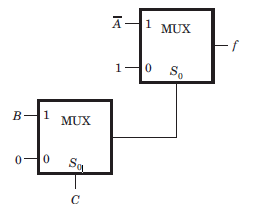Detailed Solution for Test: Combinational Logic Circuits - 1 - Question 15Test: Combinational Logic Circuits - 1 - Question 16

The MUX shown in fig. P4.2.31 is 4 * 1 multiplexer. The output Z is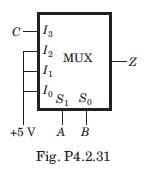Detailed Solution for Test: Combinational Logic Circuits - 1 - Question 16

Explanation : Z = (bar AB)C + (bar A)B + (bar B)A + AB

= (bar A)[(barB)C + B) + A[(bar B) + B]

= (bar A)[(B + C)] + A

= A + B + C

Test: Combinational Logic Circuits - 1 - Question 17

The output of the 4 x 1 multiplexer shown in fig. is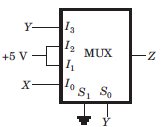Test: Combinational Logic Circuits - 1 - Question 18

The MUX shown in fig. is a 4 x 1 multiplexer. The output Z is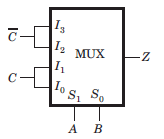Detailed Solution for Test: Combinational Logic Circuits - 1 - Question 18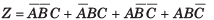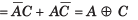Test: Combinational Logic Circuits - 1 - Question 19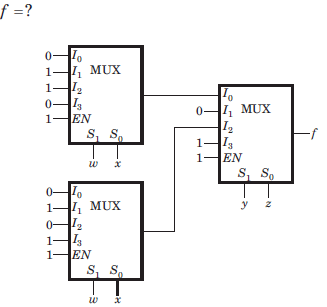Detailed Solution for Test: Combinational Logic Circuits - 1 - Question 19

The output from the upper first level multiplexer is fa and from the lower first level multiplexer is fb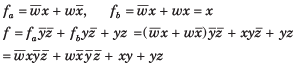Test: Combinational Logic Circuits - 1 - Question 20

For the logic circuit shown in fig.the output Y is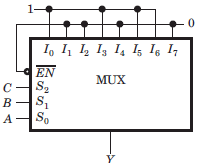Detailed Solution for Test: Combinational Logic Circuits - 1 - Question 20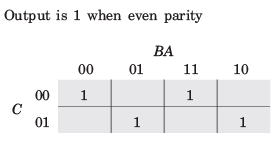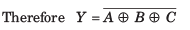## GATE ECE (Electronics) 2023 Mock Test Series

20 docs|261 tests
 Use Code STAYHOME200 and get INR 200 additional OFF Use Coupon Code
Information about Test: Combinational Logic Circuits - 1 Page
In this test you can find the Exam questions for Test: Combinational Logic Circuits - 1 solved & explained in the simplest way possible. Besides giving Questions and answers for Test: Combinational Logic Circuits - 1, EduRev gives you an ample number of Online tests for practice

## GATE ECE (Electronics) 2023 Mock Test Series

20 docs|261 tests